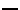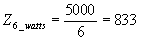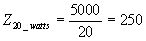## 70-Volt Loud-Speaker Matching Systems

 The EIA 70.7 volt constant voltage system of power distribution provides the engineer and technician with  a simple means of matching a number of loudspeakers to an amplifier.   To use this method: Since the voltage at rated amplifier power is 70.7, this reduces to:(2) 1.  Determine the power required at each                 loudspeaker.  2.  Add the power required for the individual      speakers and select and amplifier with a rated      power output equal to or greater than this total.  3.  Select 70.7-volt transformer having primary     wattage taps as determined in step 1.*  4.  Wire the selected primaries in parallel across      the 70.7-volt line From formula (2) these relationships are:    1 watt requires 5000 ohm primary    2 watt requires 2500 ohm primary    5 watt requires 1000 ohm primary    10 watt requires 500 ohm primary      Once the primary taps have been determined, continue on through step 4 and 5 as outlined above.  When selecting transformer primary taps, use the next highest available value above the computed value.  A  mismatch of 25% is generally considered permissible.   Example:  Required 5. Connect each secondary to its speaker; selecting     the tap which matches the voice coil inpedance. One 6 watt speaker with 4 ohm voice coil.  Two 10 watt speakers with 8 ohm voice coils    (use one transformer at this location). For transformers rated in impedance, the  following formulas may be used to determine   the proper taps in step 3. (1-2) Total power = 6 + 10 + 10 = 26 watts           (use 30-watt amplifier or other amplifier            capable of handling at least 26 watts). Primary(Amplifier output voltage)2 Impedance.Desired speaker power (3)ohms            (use 1000 ohm transformer)ohms or(1) *These transformers have the primary taps marked   in watts and the secondaries marked in ohms. (4-5) See sketch below.